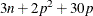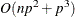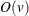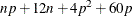# The ROBUSTREG Procedure

### Computational Resources

The algorithms for the various estimation methods need a different amount of memory for working space. Let p be the number of parameters that are estimated, and let n be the number of observations that are used in the model estimation.

For M estimation, the minimum required working space (in bytes) isIf sufficient space is available, the input data set is also kept in memory; otherwise, the input data set is read again to compute the iteratively reweighted least squares estimates, and the execution time of the procedure increases substantially. For each of the reweighted least squares,multiplications and additions are required for computing the crossproduct matrix and its inverse. Thenotation means that, for large values of the argument, v,is approximately a constant times v.

Because the iteratively reweighted least squares algorithm converges very quickly (usually within fewer than 20 iterations), the computation of M estimates is fast.

LTS estimation is more expensive in computation. The minimum required working space (in bytes) isThe memory is mainly used to store the current data that the LTS algorithm uses for modeling. The LTS algorithm uses subsampling and spends much of its computing time on resampling and computing estimates for subsamples. Because it resamples if singularity is detected, the LTS algorithm might take more time if the data set has serious singularities.

The MCD algorithm for leverage-point diagnostics is similar to the LTS algorithm.